## Example Questions

2 Next →

### Example Question #11 : Working With Function Notation

If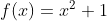and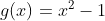, what is the value of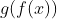?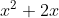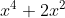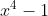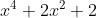Explanation:

As with many functions problems, this problem is testing whether you can follow directions. You are given two functions and then asked for the value of a nested function,. Remember when you are evaluating functions, that you must always start from the innermost function and work outward since you must always follow the order of operations. So, in this case, the problem is asking you to find the value of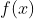and then to plug the resulting value into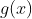.

Plugging in foris simple: you simply take whatever is the parentheses (in this case,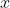) and plug it in forin the function. Your function then transforms fromto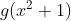.

Your next step will be similar: you just need to take what is in parentheses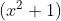and plug that value into the function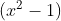every place you see anThis means thatbecomes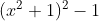. notice that the entire value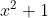is plugged into the function, not just. If you expand this function using your knowledge of perfect squares, it becomes: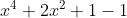, which in turn becomes.

Notice that you could also plug in a number for. If you plug in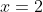, thebecomes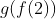. As above, remember to start from the inside and work out.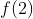becomes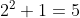The functioncan then be rewritten as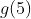. If you then plug in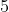to the function, you get: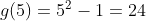.

In order to finish this problem, you simply need to plug your original value for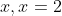into each answer choice, and look for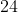.

### Example Question #11 : Working With Function Notation

If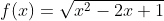, what is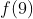?

-6

9

5

8

8

Explanation:

Function questions tend to derive most of their difficulty from the abstract function notation itself. So being comfortable with approaching function notation is most of the battle. When you see function notation such as, keep in mind thatis the "input" (whatever they tell youis, put that into the equation), and thatis the "output" (once you've put your input through the equation, the result is the value of).

So when you're given, what the problem is really saying is that "whatever we put in the parentheses of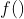, plug that value in wherever you see anin. Which means you'll take the input value, square it, subtract the product of the input value and two, add one to that, and then take the square root of the whole thing. With 9, that looks like: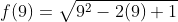You can then simplify the math underneath the radical to get: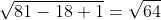And since you know that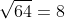you have your answer.

2 Next →

### All SAT Mathematics Resources